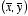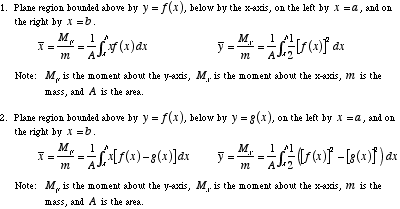# center of mass formula

Center of Mass Formula

The coordinatesof the center of mass of a plane figure are given by the formulas below. The formulas only apply for figures of uniform (constant) density.